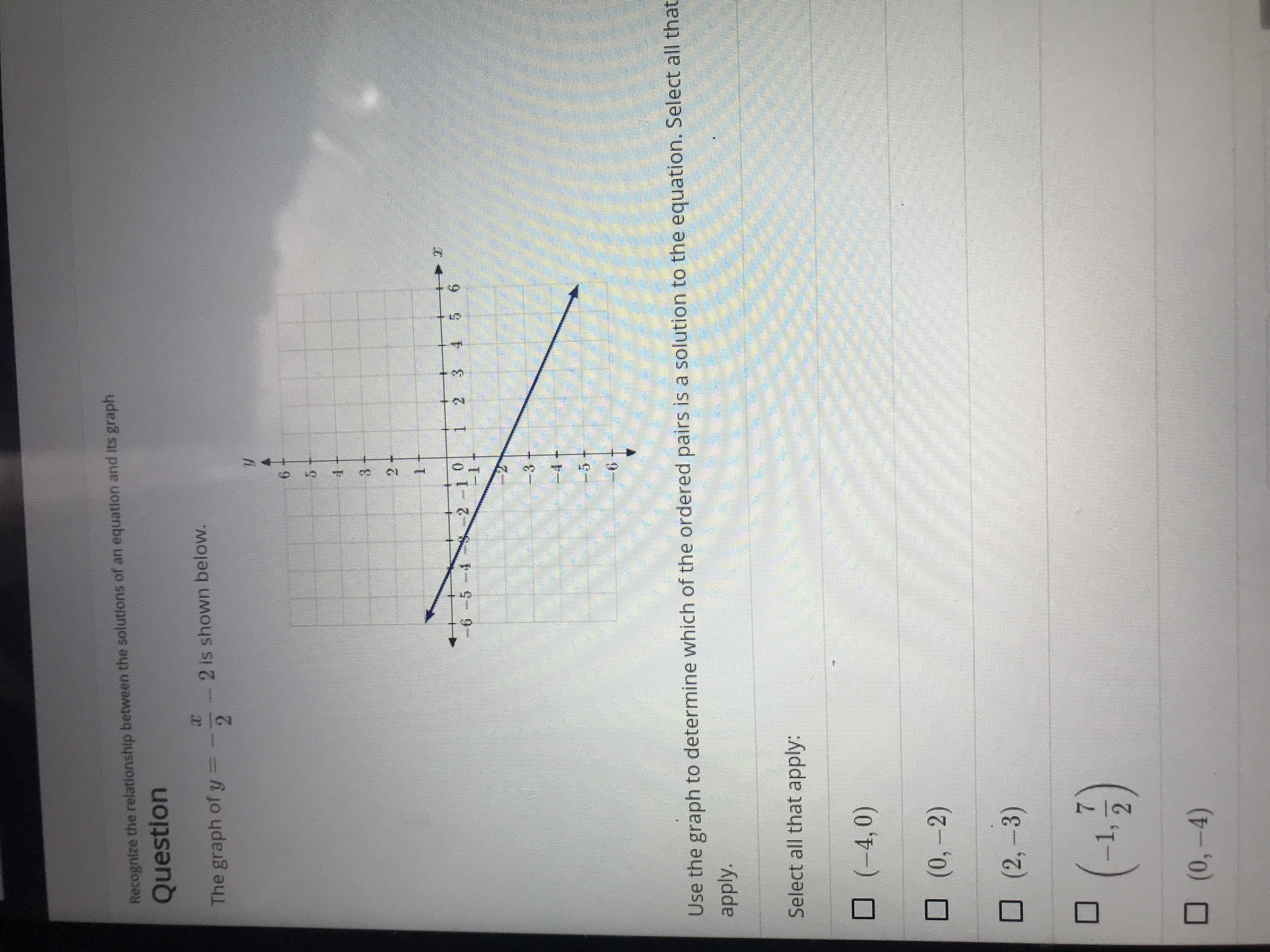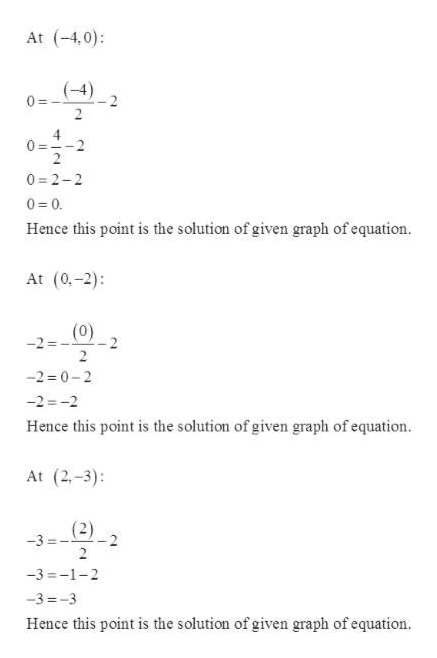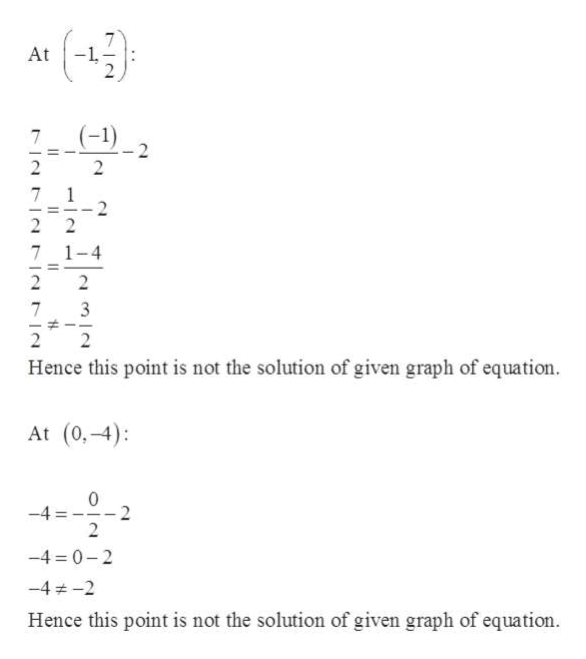# Recognize the relationship between the solutions of an equation and its graphQuestion2 is shown below.The graph of y =6532-106542 316 -5-4जभीUse the graph to determine which of the ordered pairs is a solution to the equation. Select all thatapply.Select all that apply:(-4,0)(0,-2)(2,-3)2(0,-4)- 1a

Question
86 viewshelp_outlineImage TranscriptioncloseRecognize the relationship between the solutions of an equation and its graph Question 2 is shown below. The graph of y = 6 5 3 2-10 6 5 4 2 3 1 6 -5-4 जभी Use the graph to determine which of the ordered pairs is a solution to the equation. Select all that apply. Select all that apply: (-4,0) (0,-2) (2,-3) 2 (0,-4) - 1a fullscreen
check_circle

Step 1

Given:

Step 2

Now to check whether the given points are solution or not:help_outlineImage TranscriptioncloseAt (-4,0) (-4) 0= 0--2 2 = 0 2-2 0 0 Hence this point is the solution of given graph of equation At (0,-2) (0) -2- 2 -2 0-2 -2 -2 Hence this point is the solution of given graph of equation. At (2-3) (2) -3 2 -3-1-2 -3-3 Hence this point is the solution of given graph of equation fullscreen
Step 3

Further solving for the r...help_outlineImage TranscriptioncloseAt 7 2 2 1 - - 2 2 7 1-4 2 2 7 3 2 Hence this point is not the solution of given graph of equation At (0,-4) 0 -4 2 -4 0-2 -4-2 Hence this point is not the solution of given graph of equation fullscreen

### Want to see the full answer?

See Solution

#### Want to see this answer and more?

Solutions are written by subject experts who are available 24/7. Questions are typically answered within 1 hour.*

See Solution
*Response times may vary by subject and question.
Tagged in

### Algebra